Courses

# NCERT Exemplar - Atoms and Molecules (part-1) Class 9 Notes | EduRev

## Class 9 : NCERT Exemplar - Atoms and Molecules (part-1) Class 9 Notes | EduRev

The document NCERT Exemplar - Atoms and Molecules (part-1) Class 9 Notes | EduRev is a part of the Class 9 Course Science Class 9.
All you need of Class 9 at this link: Class 9

Q.1. Which of the following represents a correct chemical formula? Name it.
(a) CaCI
(b) BiP04
(c) NaS04
(d) NaS
Ans.
(b)
BiPO4, is correct formula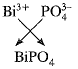Bismuth phosphate is the name of the compound.

Q.2. Write the molecular formulae for the following compounds:
(a) Copper (II) bromide
(b) Aluminium (III) nitrate
(c) Calcium (II) phosphate
(d) Iron (III) sulphide
(e) Mercury (II) chloride
(f) Magnesium (II) acetate
Ans.
(a) CuBr2
(b) Al(N03)3
(c) Ca3(PO4)2
(d) Fe2S3
(e) HgCl2
(f) (CH3COO)2Mg

Q.3. Write the molecular formulae of all the compounds that can be formed by the combination of following ions: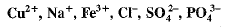Ans.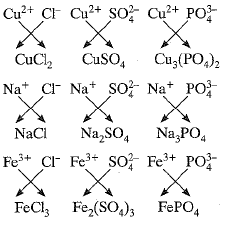Q.4. Write the cations and anions present (if any) in the following compounds:
(a) CH3COONa
(b) NaCI
(c) H2
(d) NH4NO3
Ans.
(a) CH3COO- (Anion) and Na+ (Cation)
(b) Na+ (Cation) and Cl- (Anion)
(c) H2 (No cation and No anion)
(d) NH4+ (Cation) NO3- (Anion)

Q.5. Give the formulae of the compounds formed from the following sets of elements:
(a) Calcium and fluorine
(b) Hydrogen and sulphur
(c) Nitrogen and hydrogen
(d) Carbon and chlorine
(e) Sodium and oxygen
(f) Carbon and oxygen
Ans.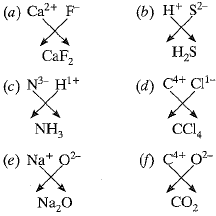Q.6. Which of the following symbols of elements are incorrect? Give their correct symbols
(a) Cobalt CO
(b) Carbon c
(c) Aluminium AL
(d) Helium He
(e) Sodium So
Ans.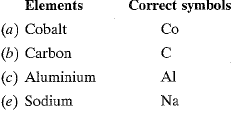Q.7. Give the chemical formulae for the following compounds and compute the ratio by mass of the combining elements in each one of them.
(a) Ammonia
(b) Carbon monoxide
(e) Hydrogen chloride
(d) Aluminium fluoride
(e) Magnesium sulphide
Ans.
(a) NH3
(b) CO
(c) HCI
(d) AlF3
(e) MgS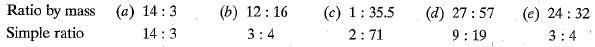Q.8. State the number of atoms present in each of the following chemical species:
(a) CO32-
(b) PO43-
(c) P2O5
(d) CO
Ans.
(a) CO32-  contains 4 atoms
(b) PO43- contains 5 atoms
(c) P2O5 contains 7 atoms
(d) CO contains 2 atoms.

Q.9. What is the fraction of the mass of water due to neutrons?
Ans.
No. of neutrons in H2O = 8 [because hydrogen does not have neutron and oxygen has 8 neutrons] Therefore, fraction of the mass of water due to neutrons =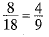Q.10. Does the solubility of a substance change with temperature? Explain with the help of an example.
Ans.
Yes, the solubility of solid in liquid increases with increase in temperature, e.g. cold water can dissolve less amount of sugar, whereas hot water can dissolve more amount of sugar.

Q.11. Classify each of the following on the basis of their atomicity.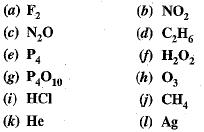Ans.
(a) Diatomic
(b) Triatomic
(c) Triatomic
(d) Octa-atomic
(e) Tetra-atomic
(f) Tetra-atomic
(g) Tetra deca-atomic (14)
(h) Triatomic
(i) Diatomic
(j) Penta-atomic
(k) Monoatomic
(l) Monoatomic

Q.12. You are provided with a fine white coloured powder which is either sugar or salt. How would you identify it without testing?
Ans.
Heat the given substance. If it turns black on heating, then it is sugar, otherwise salt because sugar will lose water on heating and black coloured carbon will be left back.
OR
Take ice cold water. If the given substance dissolves easily, it is salt. If it does not dissolve easily, it is sugar.

Q.13. Calculate the number of moles of magnesium present in a magnesium ribbon weighing 12 g. Molar atomic mass of magnesium is 24 g mol-1.
Ans.
1 mole of Mg = 24 g
24 g of Mg = 1 mole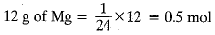14. Verify by calculating that
(a) 5 moles of C02 and 5 moles of H2O do not have the same mass.
(b) 240 g of calcium and 240 g magnesium elements have a mole ratio of 3:5:
Ans.
(a) 1 mole of CO2 = 44 g
5 moles of CO2 = 44 x 5 = 220 g
1 mole of H20 = 18 g
5 moles of H20 = 18 x 5 = 90 g
Clearly, both do not have the same mass.
(b) 1 mole of Ca = 40 g
40 g of Ca = 1 mole
240 g of Ca = 1/40 x 240 = 6 moles
24 g of Mg = 1 mole
240 g of Mg = 1/24 x 240 = 10 moles
Molar ratio = 6 : 10, i.e. 3 : 5. Hence proved.

Q.15. Find the ratio by mass of the combining elements in the following compounds:
(a) CaCO3
(b) MgCl2
(c) H2SO4
(d) C2H5OH
(e) NH3
(f) Ca(OH)
Ans.
(a) CaCO3 (b) MgCl2 (c) H2SO4 (d) C2H6O (e) NH3 (f) Ca(OH)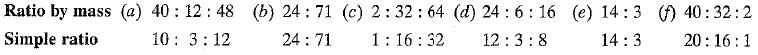Offer running on EduRev: Apply code STAYHOME200 to get INR 200 off on our premium plan EduRev Infinity!

## Science Class 9

95 videos|223 docs|135 tests

,

,

,

,

,

,

,

,

,

,

,

,

,

,

,

,

,

,

,

,

,

;